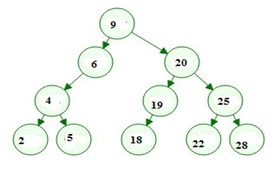# Question & Answer: Follow the TREE-DELETE procedure described in the textbook, show step-by-step…..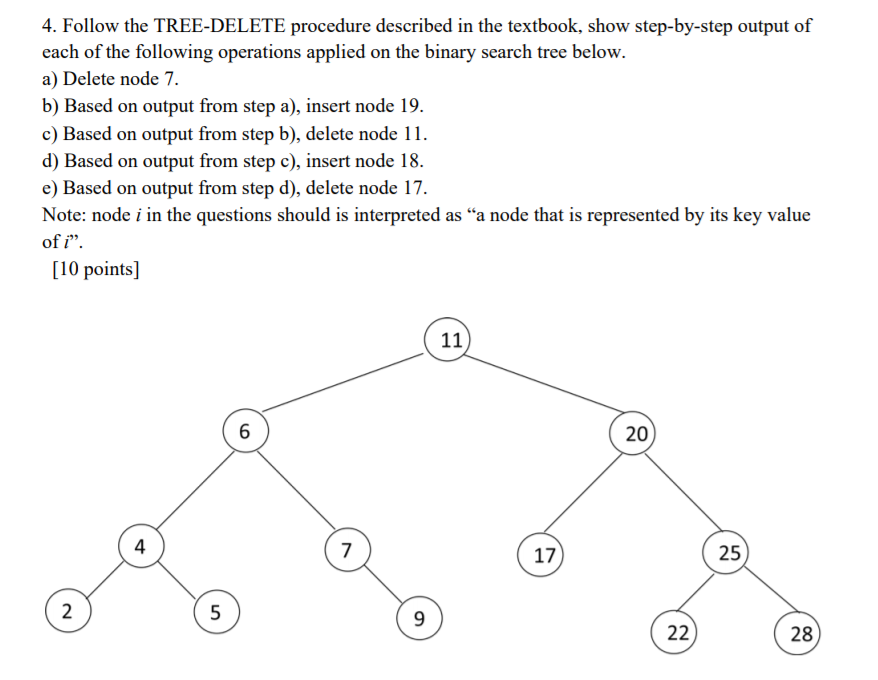Follow the TREE-DELETE procedure described in the textbook, show step-by-step output of each of the following operations applied on the binary search tree below. a) Delete node 7. b) Based on output from step a), insert node 19. c) Based on output from step b), delete node 11. d) Based on output from step c), insert node 18. e) Based on output from step d), delete node 17.

Don't use plagiarized sources. Get Your Custom Essay on
Question & Answer: Follow the TREE-DELETE procedure described in the textbook, show step-by-step…..
GET AN ESSAY WRITTEN FOR YOU FROM AS LOW AS \$13/PAGE

Step1:

a)delete node 7

7< 11 :looking at left subtree

7>6 :looking at right subtree

7==7 :found the node to delete

node to delete has no left child set parent of deleted node to right child of delete node.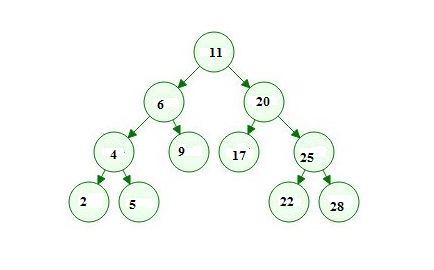Step2:insert node 19

19>11 looking at right sub tree

19<20 looking at left sub tree

19>17 looking at right sub tree

found null tree insert element.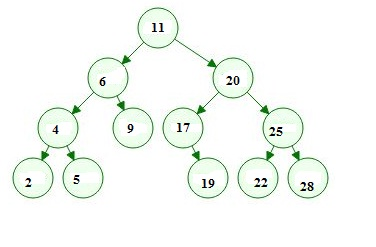step3:delete 11

11==11 found node to delete.

node to delete has two children’s find the largest node in left sub treestep4:insert 18

18>9 looking at right sub tree

18<20 looking at left subtree

18>17 looking at right subtree

18<19 looking at left subtree

found null insert left to 19.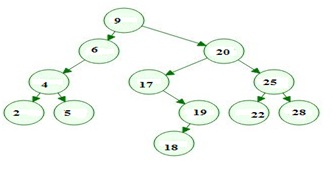step5: delete17

17>9 looking at right sub tree

17<20 looking at left sub tree

17==17 founf node to delete

node to delete has no left child

set parent of deleted node to right child of deleted node.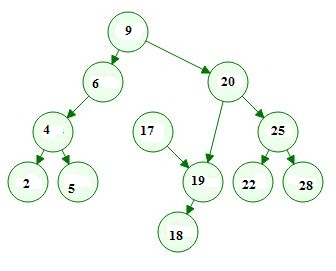final tree: